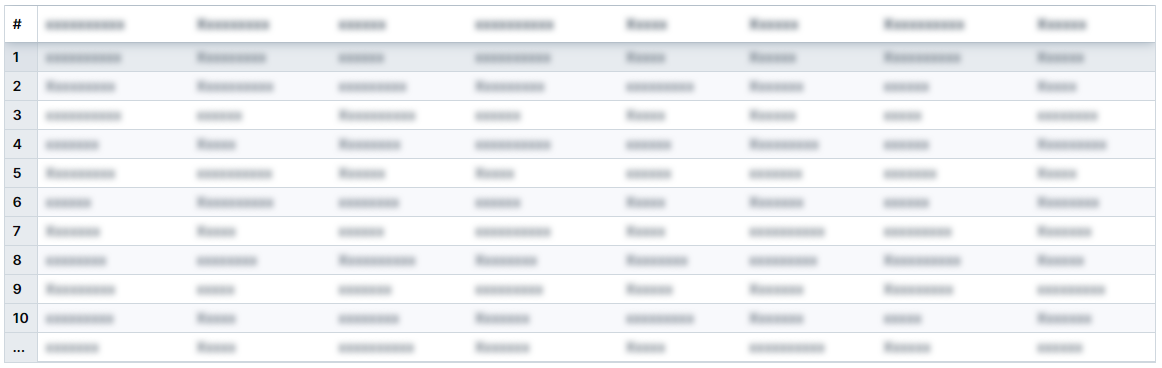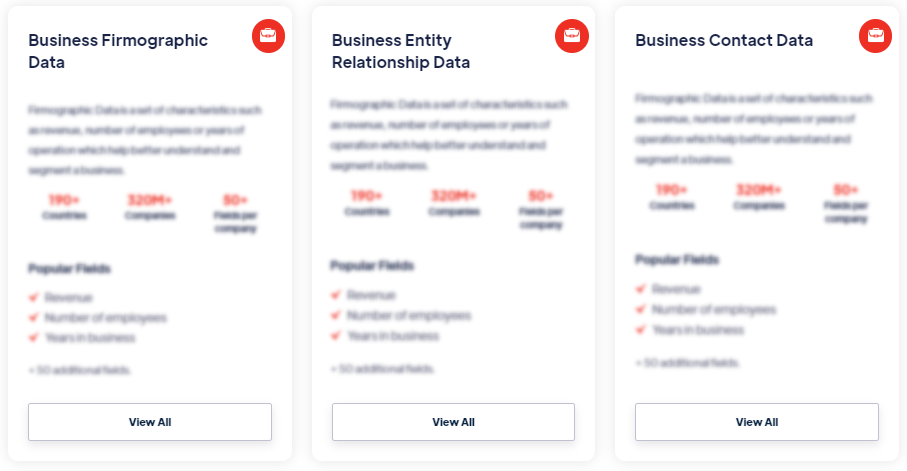# Products

Regression is a statistical method used to examine the relationship between a dependent variable and one or more independent variables. It aims to model the relationship between the variables and make predictions or estimations based on that relationship. Read more

#### Our Data Integrations### Regression### Browse the Data Marketplace1. What is Regression?
Regression is a statistical method used to examine the relationship between a dependent variable and one or more independent variables. It aims to model the relationship between the variables and make predictions or estimations based on that relationship.

2. Why is Regression important?
Regression analysis is widely used in various fields, including economics, finance, social sciences, and machine learning. It helps in understanding the nature of the relationship between variables, predicting future outcomes, identifying significant factors, and making informed decisions based on data analysis.

3. What are the types of Regression?
There are several types of regression analysis techniques, including simple linear regression, multiple linear regression, polynomial regression, logistic regression, and more. The choice of regression technique depends on the nature of the data and the relationship being examined.

4. How does Regression work?
Regression models the relationship between the dependent variable and independent variables by estimating coefficients that represent the impact of the independent variables on the dependent variable. The model equation is derived using statistical methods to minimize the difference between the observed and predicted values.

5. What are the key components of a Regression model?
A regression model typically includes a dependent variable, independent variables, regression coefficients, an error term, and an equation that represents the relationship between the variables. The model is built based on the assumption that the relationship is linear, and certain assumptions about the errors are met.

6. How is Regression used in practice?
Regression analysis is applied in various ways, such as predicting sales based on advertising expenditure, estimating the impact of variables on stock prices, determining the relationship between variables in social sciences, and developing predictive models in machine learning.

7. What are the limitations of Regression?
Regression analysis has certain limitations, such as assuming a linear relationship between variables, potential presence of multicollinearity (high correlation between independent variables), sensitivity to outliers, and the need to meet certain assumptions for accurate results. Careful interpretation and validation of the model are crucial to ensure reliable insights.